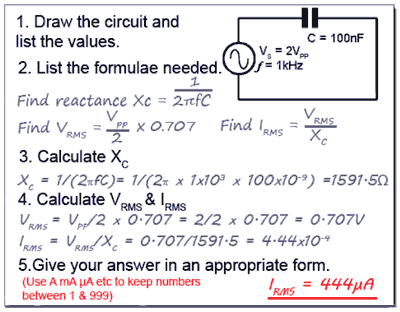# Capacitive Reactance (XC) Calculations

• After studying this section, you should be able to describe:
• • Calculations involving capacitive reactance.
• • Multi stage calculations involving reactance & resistance.

For calculations based on Capacitive Reactance, you firstly need to think about the information on the "Reactance & Resistance page" and appreciate the differences between reactance and resistance and the two types of reactance. For calculations you can choose which formula to use for capacitive reactance, either 1/2πƒC or 1/ωC, but 1/2πƒC is more commonly used, (one reason being that scientific calculators mostly have a π key, but no ω key!).

Remember that some problems you may need to work out will not necessarily have an obvious solution, such as just calculating the reactance of a component. For example, if you are asked to calculate the supply voltage required to produce a certain current flow through, or voltage across a component, two or more steps may be needed, using the answer from one calculation to provide information for a second calculation before reaching the final answer.

Before you start, think about the following tips; they will make the problems easier if you follow them carefully.

1. Work out the answers using pencil and paper; redraw the circuit you are working on.

2. List the items of information you are given, and what you need to find for your answer. Doing this will help you decide whether the answer can be found in a single step or if you will need an intermediate answer.

3. Once you have listed the information in step 2 you will need to decide on which appropriate formula (or formulae) to use. Write this down as well.

4. Of course the answer is not just a number, if it is a certain number of Ohms (or any other unit), don't forget to show the correct unit multiple (e.g. Ω, KΩ or MΩ) otherwise your answer is meaningless.

5. When you put values into your calculator convert all large or small (Meg, micro etc.) values into their basic units (volts ohms etc.) by using the EXP key. Its easy to slip up here and get really stupid answers, thousands of times too big or too small.

All these steps may seem rather a toil at first, but get into the habit and they will make your calculations easier, because you will be following a familiar method. They will also be more reliable, because when you need to carry out multiple step calculations you need to be organised. It is so easy to go wrong part way through your working out because you have forgotten just where you are in the calculation. If you have written each problem out however, it will allow you to go back and see where you went wrong so you don't keep making the same mistakes.

### Why go to all this trouble when there are lots of calculators on the web that will do the calculations for you?

Many electronic and web based calculators are excellent, just put in the data and click for the answer. But you still need to know instinctively which formula to use, and when, and why. To be familiar enough to do this well, you need to know how the various formulae work. The best way to do this is to start by working out some problems manually, then you will find many of the calculators offered on websites much more useful.To help you on the right track why not download our "Maths Tips" booklet, which shows you how to use your calculator with exponents and engineering notation to deal with these units and get the right answer every time.

No scientific calculator? The "Maths Tips" booklet explains what you need (and what you don't need so you don't spend your money unnecessarily). If you don't want to buy a scientific calculator, you can always pick up a free one from www.calculator.org/download.html. PC users can try Calc98.

Whichever calculator you choose, remember that you should read the instructions to become familiar with the working methods you should use as these do vary from calculator to calculator.

OK so now you have read these instructions, you are ready to start. Here is a way to solve a typical problem on paper so (with practice) you don't get confused.## Reactance Examples.

The problem illustrated in Fig 6.4.1 is a typical example where a number of related values need to be found, including the reactance of the capacitor. Other values, such as the RMS Voltage (VRMS) and RMS Current (IRMS) are described in Module 1.2

Note: If you are using Calc98 for your calculations you need to set the View>Option>Display menu to Engineering (under the "Decimal" choices) and it would be a good idea whilst you are in this menu to select 2 from the Decimals drop down box to set the number of digits after the decimal place. This will round your answer down to two decimal places which is sufficiently accurate for most uses and stops you getting silly answers such as 75.666666666667Ω, which would be far too accurate for most purposes.